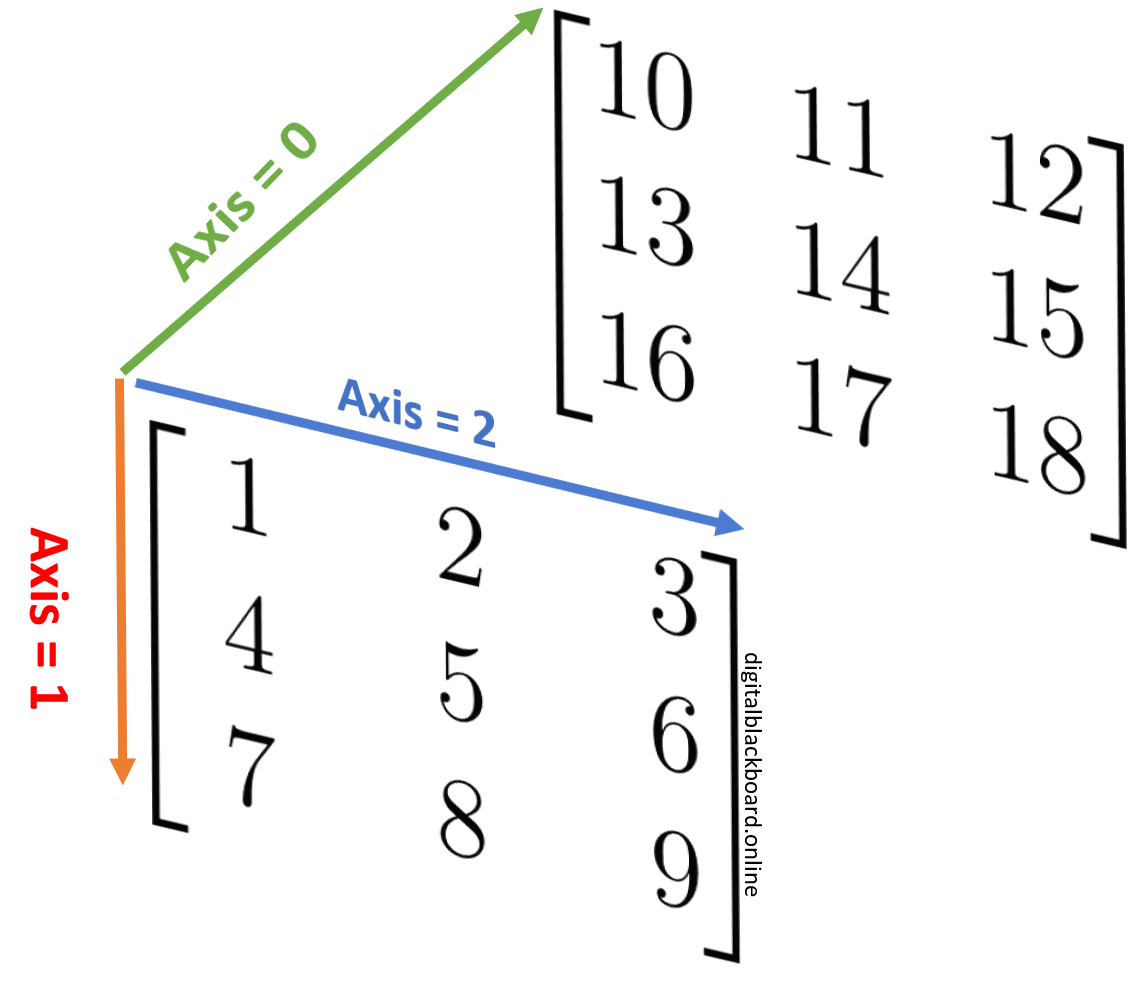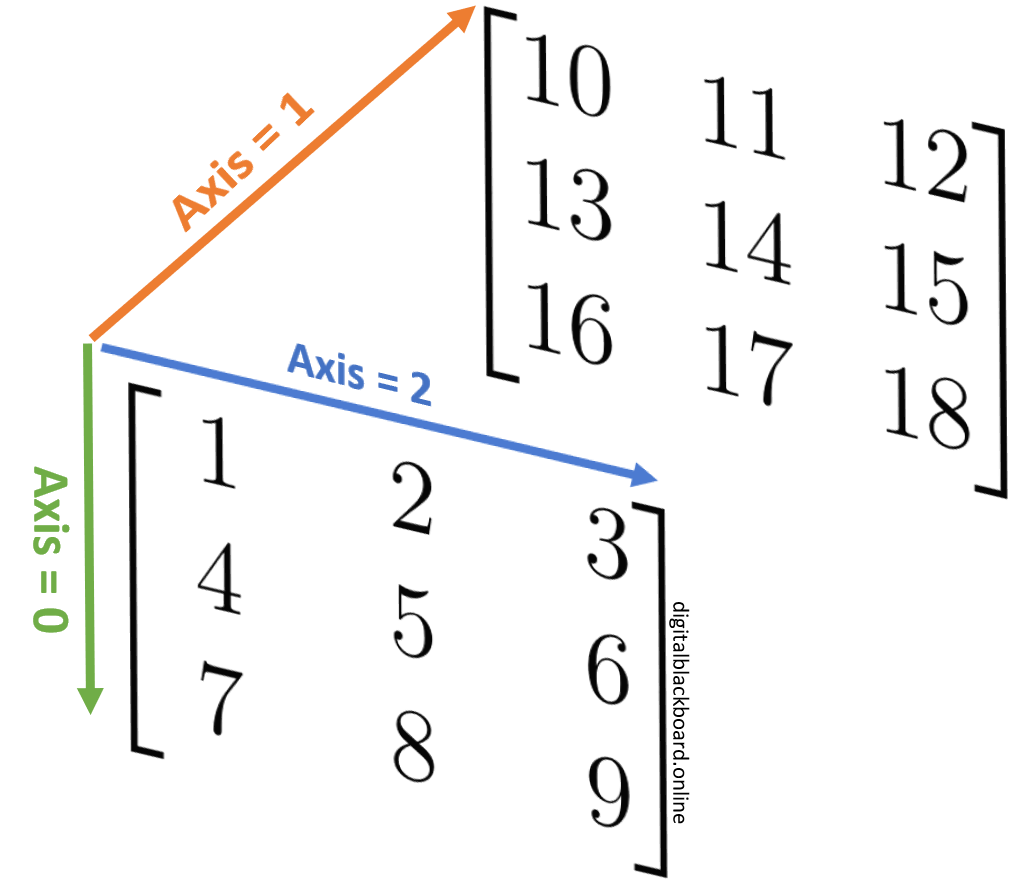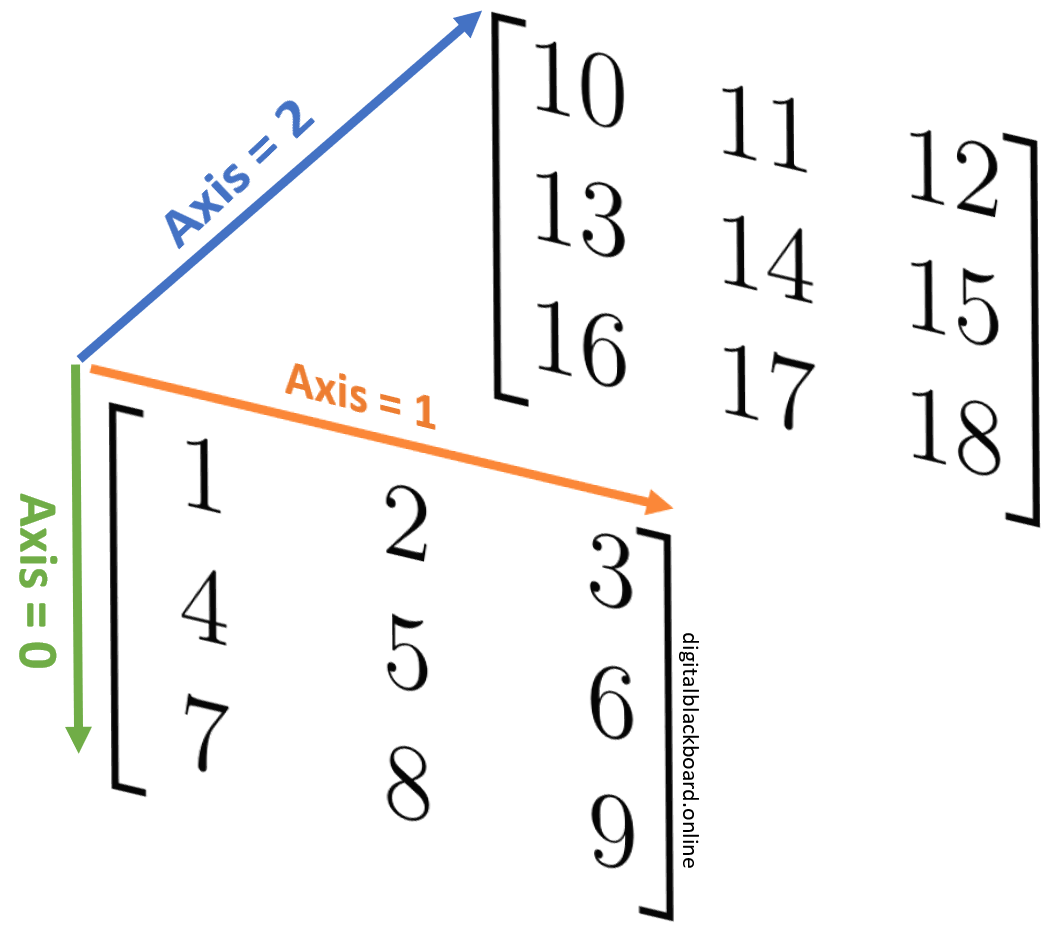## Introduction

Concatenation means joining of two or more arrays into a single array. Several functions are available in NumPy to perform concatenation including numpy.concatenate, numpy.vstack, numpy.hstack and numpy.stack.

## The numpy.concatenate Function

The numpy.concatenate function joins a sequence of arrays along an existing axis.

Syntax

The numpy.concatenate function.

1numpy.concatenate((a1, a2, ...), axis=0, out=None, dtype=None)

Parameter Required? Default Value Description
a1, a2, ... ✔️ Yes NA Input arrays must have the same shape, except in the dimension corresponding to axis.
axis ❌ No 0 The axis along which the arrays will be joined. If axis is None, arrays are flattened before use.
out ❌ No None If provided, the destination to place the result. The shape must be correct, matching that of what concatenate would have returned if no out argument were specified.
dtype ❌ No None If provided, the destination array will have this dtype. Cannot be provided together with out.
Note

All the input arrays must have same number of dimensions.

### Joining 1-D Arrays

We begin by first by creating a few arrays to be used in subsequent examples. In the following, ar is a 2-D array and x, y and z are 1-D arrays sliced from ar.

1import numpy as np
2ar = np.arange(1, 10).reshape((3, 3)) # create 2D array
3print(ar)
4x = ar[0,:] # 3 1-D arrays (each one is a row)
5print(x)
6y = ar[1,:]
7print(y)
8z = ar[2,:]
9print(z)

[[1 2 3]
[4 5 6]
[7 8 9]]

[1 2 3]

[4 5 6]

[7 8 9]


Example

Concatenating two 1-D arrays.

1np.concatenate((x, y))

array([1, 2, 3, 4, 5, 6])


Example

Concatenating three 1-D arrays.

1np.concatenate((x, y, z))

array([1, 2, 3, 4, 5, 6, 7, 8, 9])


Example

Concatenating three 1-D arrays with dtype=float.

1np.concatenate((x, y, z),dtype=float)

array([1., 2., 3., 4., 5., 6., 7., 8., 9.])

Caution

It's not possible to concatenate 1-D arrays along axis=1 (and hoping that the arrays will stack row by row) since the numpy.concatenate function only concatenates arrays along an existing axis.

### Joining 2-D Arrays

We begin by first by creating two more 2-D arrays to be used in subsequent examples.

1ar2 = np.arange(10, 19).reshape((3, 3))
2print(ar2)
3ar3 = np.arange(19, 28).reshape((3, 3))
4print(ar3)

[[10 11 12]
[13 14 15]
[16 17 18]]

[[19 20 21]
[22 23 24]
[25 26 27]]


Example

Concatenating two 2-D arrays along first axis (default).

1np.concatenate((ar2, ar3))

array([[10, 11, 12],
[13, 14, 15],
[16, 17, 18],
[19, 20, 21],
[22, 23, 24],
[25, 26, 27]])


Example

Concatenating two 2-D arrays along second axis.

1np.concatenate((ar2, ar3),axis=1)

array([[10, 11, 12, 19, 20, 21],
[13, 14, 15, 22, 23, 24],
[16, 17, 18, 25, 26, 27]])


Example

Concatenating two 2-D arrays with axis=None.

1np.concatenate((ar2, ar3),axis=None)

array([10, 11, 12, 13, 14, 15, 16, 17, 18, 19, 20, 21, 22, 23, 24, 25, 26, 27])


In the above, the input arrays are flattened before concatenation.

Example

Concatenating three 2-D arrays along second axis.

1np.concatenate((ar, ar2, ar3), axis=1)

array([[ 1,  2,  3, 10, 11, 12, 19, 20, 21],
[ 4,  5,  6, 13, 14, 15, 22, 23, 24],
[ 7,  8,  9, 16, 17, 18, 25, 26, 27]])


Example

Concatenate a 1-D array with a 2-D array results in error.

1np.concatenate((x, ar2))

File "<__array_function__ internals>", line 5, in concatenate

ValueError: all the input arrays must have same number of dimensions, but the array at index 0 has 1 dimension(s) and the array at index 1 has 2 dimension(s)

Caution

All the input arrays must have same number of dimensions.

However, it is still possible to concatenate arrays with different dimensions. For the above example, one way is to convert the 1-D array into a 2-D array using either the newaxis method or reshape() method.

Example

Concatenate a 1-D array with a 2-D array by using newaxis.

1np.concatenate((x[np.newaxis, :], ar2))

array([[ 1,  2,  3],
[10, 11, 12],
[13, 14, 15],
[16, 17, 18]])


Example

Concatenate a 1-D array with a 2-D array by using reshape() method.

1np.concatenate((x.reshape(1,3), ar2))

array([[ 1,  2,  3],
[10, 11, 12],
[13, 14, 15],
[16, 17, 18]])


## numpy.vstack: Joining Arrays Vertically

The numpy.vstack function stacks arrays in sequence vertically (row wise). In contrast to the numpy.concatenate function, it is possible to stack two 1-D arrays along the second axis which would result in putting them one on top of the other, or even stacking arrays of different dimensions.

Example

Stack two 1-D arrays vertically using numpy.vstack.

1np.vstack((x, y))

array([[1, 2, 3],
[4, 5, 6]])


Example

Stack two 2-D arrays vertically using numpy.vstack.

1np.vstack((ar, ar2))

array([[ 1,  2,  3],
[ 4,  5,  6],
[ 7,  8,  9],
[10, 11, 12],
[13, 14, 15],
[16, 17, 18]])


Example

Stack two arrays (of different dimensions) vertically using numpy.vstack.

1np.vstack((x, ar2))

array([[ 1,  2,  3],
[10, 11, 12],
[13, 14, 15],
[16, 17, 18]])


## numpy.hstack: Joining Arrays Horizontally

The numpy.hstack function stacks arrays in sequence horizontally (column wise). This is equivalent to concatenation along the second axis, except for 1-D arrays where it concatenates along the first axis.

Note

The arrays must have the same shape along all but the second axis, except 1-D arrays which can be any length.

Example

Stack two 1-D arrays horizontally using numpy.hstack.

1np.hstack((x, y))

array([1, 2, 3, 4, 5, 6])


The above can also be reproduced using np.concatenate((x, y)).

Example

Stack two 2-D arrays horizontally using numpy.hstack.

1np.hstack((ar, ar2))

array([[ 1,  2,  3, 10, 11, 12],
[ 4,  5,  6, 13, 14, 15],
[ 7,  8,  9, 16, 17, 18]])


The above can also be reproduced using np.concatenate((ar, ar2), axis=1).

Tip

The advantage of using numpy.hstack over numpy.concatenate is that there is no need to specify the axis parameter.

It is possible to stack two arrays of different shapes horizontally as long as the they have the same size along the interface. In other words, the arrays must have the same shape along all but the second axis.

Example

Stack two arrays (of different shapes) horizontally using numpy.hstack.

1ar4 = np.arange(0, 15).reshape((3, 5)) # create a 3X5 array
2print(ar4)
3print(np.hstack((ar2, ar4)))

[[ 0  1  2  3  4]
[ 5  6  7  8  9]
[10 11 12 13 14]]

[[10 11 12  0  1  2  3  4]
[13 14 15  5  6  7  8  9]
[16 17 18 10 11 12 13 14]]


It is meaningless to stack two arrays that do not have the same size along the interface. Instead, we may reshape one of the arrays to ensure they can be stacked properly.

Example

Stack two (unmatched) arrays horizontally using numpy.hstack.

1np.hstack((x.reshape(3,1), ar2))

array([[ 1, 10, 11, 12],
[ 2, 13, 14, 15],
[ 3, 16, 17, 18]])


As another example, we can extract a single row from the 2-D array ar2 so that it can then be stacked horizontally with the 1-D array x.

Example

Stack two (unmatched) arrays horizontally using numpy.hstack.

1np.hstack((x, ar2[0,:] ))

array([ 1,  2,  3, 10, 11, 12])


## numpy.stack: Joining Arrays Along a New Axis

While the concatenate, hstack and vstack functions join arrays along an existing axis, the numpy.stack function joins a sequence of arrays along a new axis.

Syntax

The numpy.stack function.

1numpy.stack((a1, a2, ...), axis=0, out=None)

Parameter Required? Default Value Description
a1, a2, ... ✔️ Yes NA Each array must have the same shape.
axis ❌ No 0 The axis in the result array along which the input arrays are stacked.
out ❌ No None If provided, the destination to place the result. The shape must be correct, matching that of what stack would have returned if no out argument were specified.

The axis parameter specifies the index of the new axis in the dimensions of the result. For example, if axis=0 it will be the first dimension and if axis=-1 it will be the last dimension.

Example

Stack two 2-D arrays along axis=0 using numpy.stack.

1np.stack((ar, ar2),axis=0) # size=2 along axis=0

array([[[ 1,  2,  3],
[ 4,  5,  6],
[ 7,  8,  9]],

[[10, 11, 12],
[13, 14, 15],
[16, 17, 18]]])

1np.stack((ar, ar2),axis=0).shape # output shape

(2, 3, 3)Note

The size along the axis that is stacked is always equal to the number of input arrays.

In the above example, there are two input arrays which are stacked along axis=0. Therefore, the size of the output array for axis=0 (first dimension) will also be two.

Example

Stack two 2-D arrays along axis=1 using numpy.stack.

1np.stack((ar, ar2),axis=1) # size=2 along axis=1

array([[[ 1,  2,  3],
[10, 11, 12]],

[[ 4,  5,  6],
[13, 14, 15]],

[[ 7,  8,  9],
[16, 17, 18]]])

1np.stack((ar, ar2),axis=1).shape # output shape

(3, 2, 3)In this case, there are two input arrays which are stacked along axis=1 (second dimension). Therefore, the size of the output array for the second dimension will also be two.

Tip

An easy way to visualize stacking is to simply swap the axes so that the input arrays are stacked along the specified axis.

Example

Stack two 2-D arrays along axis=2 using numpy.stack.

1np.stack((ar, ar2),axis=2) # size=2 along axis=2

array([[[ 1, 10],
[ 2, 11],
[ 3, 12]],

[[ 4, 13],
[ 5, 14],
[ 6, 15]],

[[ 7, 16],
[ 8, 17],
[ 9, 18]]])

1np.stack((ar, ar2),axis=2).shape # output shape

(3, 3, 2)In this case, there are two input arrays which are stacked along axis=2 (third dimension). Therefore, the size of the output array for the third dimension will also be two.